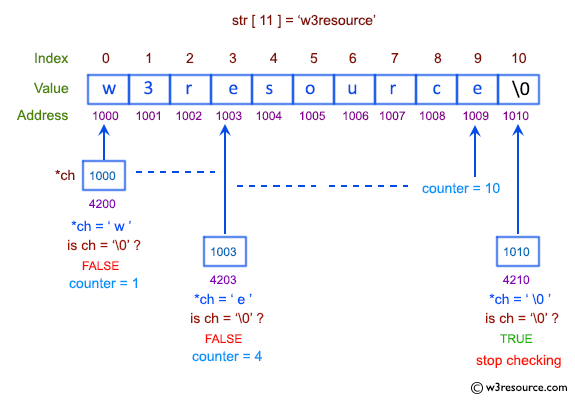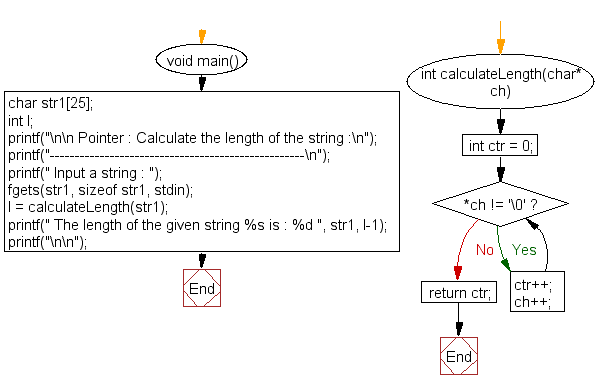﻿ C Program: Calculate the length of the string - w3resource# C Exercises: Calculate the length of the string

## C Pointer : Exercise-10 with Solution

Write a program in C to Calculate the length of the string using a pointer.

Pictorial Presentation:Sample Solution:

C Code:

``````#include <stdio.h>
int calculateLength(char*);

void main()
{
char str1;
int l;
printf("\n\n Pointer : Calculate the length of the string :\n");
printf("---------------------------------------------------\n");

printf(" Input a string : ");
fgets(str1, sizeof str1, stdin);

l = calculateLength(str1);
printf(" The length of the given string %s is : %d ", str1, l-1);
printf("\n\n");

}

int calculateLength(char* ch) // ch = base address of array str1 ( &str1  )
{
int ctr = 0;
while (*ch != '\0')
{
ctr++;
ch++;
}
return ctr;
}
```
```

Sample Output:

``` Pointer : Calculate the length of the string :
---------------------------------------------------
Input a string : w3resource
The length of the given string w3resource
is : 10
```

Flowchart:C Programming Code Editor:

Have another way to solve this solution? Contribute your code (and comments) through Disqus.

What is the difficulty level of this exercise?

Test your Programming skills with w3resource's quiz.

﻿

## C Programming: Tips of the Day

How to format strings using printf() to get equal length in the output?

You can specify a width on string fields, e.g.

`printf("%-20s", "initialization..."); `

And then whatever's printed with that field will be blank-padded to the width you indicate.

The - left-justifies your text in that field.

Ref : https://bit.ly/34DMOc3